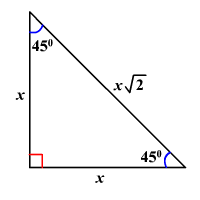# 45°-45°-90° Triangles

There is a special relationship among the measures of the sides of a $45°-45°-90°$ triangle.

$45°-45°-90°$ triangle is a commonly encountered right triangle whose sides are in the proportion $1:1:\sqrt{2}$ . The measures of the sides are $x$ , $x$ , and $x\sqrt{2}$ .In a $45°-45°-90°$ triangle, the length of the hypotenuse is $\sqrt{2}$ times the length of a leg.

To see why this is so, note that by the Converse of the Pythagorean Theorem , these values make the triangle a right triangle.

$\begin{array}{l}{x}^{2}+{x}^{2}=2{x}^{2}\\ \text{\hspace{0.17em}}\text{\hspace{0.17em}}\text{\hspace{0.17em}}\text{\hspace{0.17em}}\text{\hspace{0.17em}}\text{\hspace{0.17em}}\text{\hspace{0.17em}}\text{\hspace{0.17em}}\text{\hspace{0.17em}}\text{\hspace{0.17em}}\text{\hspace{0.17em}}\text{\hspace{0.17em}}\text{\hspace{0.17em}}\text{\hspace{0.17em}}\text{\hspace{0.17em}}\text{\hspace{0.17em}}={\left(x\sqrt{2}\right)}^{2}\end{array}$

Note that an isosceles right triangle must be a $45°-45°-90°$ triangle.